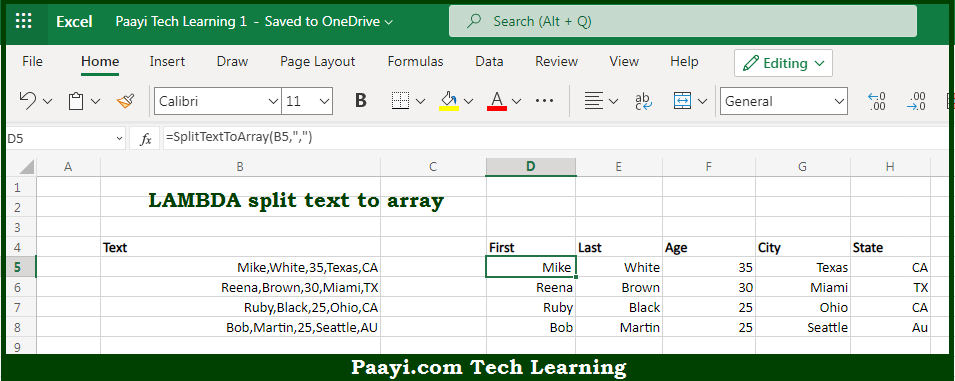# Learn How to LAMBDA Split Text to Array in Microsoft Excel

Written by | 0 Comments | 626 Views

In this article, you will learn how to evaluate things in Dynamic Arrays with formulas in Microsoft Excel using a single/combination(s) of functions. You will also know How to LAMBDA Split Text to Array and see the generic formula.

Learn How to LAMBDA Split Text to Array in Microsoft Excel

The main purpose of this formula is to split the text into arrays. Here we will learn how to split text to array in the given data range in the workbook in Microsoft Excel. That implies, with the help of a formula based on the LAMBDA function you can able to split the text into arrays. So, with the help of this formula, you can able text to array in the given data range in the workbook in Microsoft Excel.

General Formula to LAMBDA Split Text to Array

=LAMBDA(text,delim,TRANSPOSE(FILTERXML("<x><y>"&SUBSTITUTE(text,delim,"</y><y>")&"</y></x>","//y")))

The Explanation for LAMBDA Split Text to ArraySo we know that with the help of the given formula above you can able to split the text into arrays. Here we will learn how to split text to array in the given data range in the workbook in Microsoft Excel. As we know that the first step in creating a custom LAMBDA function is to verify the logic required in the standard formula. Here the LAMBDA formula is based on an interesting formula created with the FILTERXML, SUBSTITUTE, and TRANSPOSE functions. So, with the help of this formula, you can able to split a text into arrays. Here we will learn how to split text to array in the given data range in the workbook in Microsoft Excel.